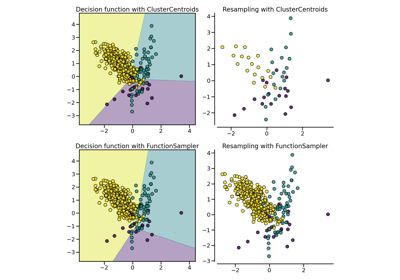# imblearn.under_sampling.InstanceHardnessThreshold¶

class imblearn.under_sampling.InstanceHardnessThreshold(*, estimator=None, sampling_strategy='auto', random_state=None, cv=5, n_jobs=None)[source]

Undersample based on the instance hardness threshold.

Read more in the User Guide.

Parameters
estimatorobject, default=None

Classifier to be used to estimate instance hardness of the samples. By default a sklearn.ensemble.RandomForestClassifier will be used. If str, the choices using a string are the following: 'knn', 'decision-tree', 'random-forest', 'adaboost', 'gradient-boosting' and 'linear-svm'. If object, an estimator inherited from sklearn.base.ClassifierMixin and having an attribute predict_proba.

sampling_strategyfloat, str, dict, callable, default=’auto’

Sampling information to sample the data set.

• When float, it corresponds to the desired ratio of the number of samples in the minority class over the number of samples in the majority class after resampling. Therefore, the ratio is expressed aswhereis the number of samples in the minority class andis the number of samples in the majority class after resampling.

Warning

float is only available for binary classification. An error is raised for multi-class classification.

• When str, specify the class targeted by the resampling. The number of samples in the different classes will be equalized. Possible choices are:

'majority': resample only the majority class;

'not minority': resample all classes but the minority class;

'not majority': resample all classes but the majority class;

'all': resample all classes;

'auto': equivalent to 'not minority'.

• When dict, the keys correspond to the targeted classes. The values correspond to the desired number of samples for each targeted class.

• When callable, function taking y and returns a dict. The keys correspond to the targeted classes. The values correspond to the desired number of samples for each class.

random_stateint, RandomState instance, default=None

Control the randomization of the algorithm.

• If int, random_state is the seed used by the random number generator;

• If RandomState instance, random_state is the random number generator;

• If None, the random number generator is the RandomState instance used by np.random.

cvint, default=5

Number of folds to be used when estimating samples’ instance hardness.

n_jobsint, default=None

Number of CPU cores used during the cross-validation loop. None means 1 unless in a joblib.parallel_backend context. -1 means using all processors. See Glossary for more details.

NearMiss

Undersample based on near-miss search.

RandomUnderSampler

Random under-sampling.

Notes

The method is based on .

Supports multi-class resampling. A one-vs.-rest scheme is used when sampling a class as proposed in .

References

1(1,2)

D. Smith, Michael R., Tony Martinez, and Christophe Giraud-Carrier. “An instance level analysis of data complexity.” Machine learning 95.2 (2014): 225-256.

Examples

>>> from collections import Counter
>>> from sklearn.datasets import make_classification
>>> from imblearn.under_sampling import InstanceHardnessThreshold
>>> X, y = make_classification(n_classes=2, class_sep=2,
... weights=[0.1, 0.9], n_informative=3, n_redundant=1, flip_y=0,
... n_features=20, n_clusters_per_class=1, n_samples=1000, random_state=10)
>>> print('Original dataset shape %s' % Counter(y))
Original dataset shape Counter({1: 900, 0: 100})
>>> iht = InstanceHardnessThreshold(random_state=42)
>>> X_res, y_res = iht.fit_resample(X, y)
>>> print('Resampled dataset shape %s' % Counter(y_res))
Resampled dataset shape Counter({1: 5..., 0: 100})

Attributes
sample_indices_ndarray of shape (n_new_samples)

Indices of the samples selected.

New in version 0.4.

__init__(self, *, estimator=None, sampling_strategy='auto', random_state=None, cv=5, n_jobs=None)[source]

Initialize self. See help(type(self)) for accurate signature.

## Examples using imblearn.under_sampling.InstanceHardnessThreshold¶